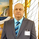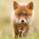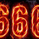10158 lượt xem
EXPERIMENTAL:
fan projection from zigzag .
```study(title='[RS]PA Zigzag Fibonacci FAN V0', shorttitle='F', overlay=true)
useHA = input(false, title='Use Heikken Ashi Candles')
useAltTF = input(true, title='Use Alt Timeframe')
tf = input('D', title='Alt Timeframe')

zigzag() =>
_isUp = close >= close
_isDown = close <= close
_direction = _isUp and _isDown ? -1 : _isDown and _isUp ? 1 : nz(_direction)
_zigzag = _isUp and _isDown and _direction != -1 ? highest(2) : _isDown and _isUp and _direction != 1 ? lowest(2) : na

_ticker = useHA ? heikenashi(tickerid) : tickerid
sz = useAltTF ? (change(time(tf)) != 0 ? security(_ticker, tf, zigzag()) : na) : zigzag()

plot(sz, title='zigzag', color=black, linewidth=2)

//  ||---   Pattern Recognition:

x = valuewhen(sz, sz, 1)
z = valuewhen(sz, sz, 0)
n_x = valuewhen(sz, n, 1)
n_z = valuewhen(sz, n, 0)

price_range = z-x
bar_range = n_z-n_x
step = price_range/bar_range
projection = sz ? na : z + ((n-n_z)*step)
projection_n0236 = sz ? na : z + ((n-n_z)*step)*-0.236
projection_n0382 = sz ? na : z + ((n-n_z)*step)*-0.382
projection_n0500 = sz ? na : z + ((n-n_z)*step)*-0.500
projection_n0618 = sz ? na : z + ((n-n_z)*step)*-0.618
projection_n1000 = sz ? na : z + ((n-n_z)*step)*-1.000
projection_n1618 = sz ? na : z + ((n-n_z)*step)*-1.618
projection_n3141 = sz ? na : z + ((n-n_z)*step)*-3.141
plot(title='0.0', series=projection, style=linebr, color=blue)
plot(title='-0.236', series=projection_n0236, style=linebr, color=gray)
plot(title='-0.382', series=projection_n0382, style=linebr, color=yellow)
plot(title='-0.500', series=projection_n0500, style=linebr, color=orange)
plot(title='-0.618', series=projection_n0618, style=linebr, color=red)
plot(title='-1.000', series=projection_n1000, style=linebr, color=black)
plot(title='-1.618', series=projection_n1618, style=linebr, color=maroon)
plot(title='-3.141', series=projection_n3141, style=linebr, color=aqua)
```

## Bình luậnHi Ricardo:

Where the variable n comes from in: n_z = valuewhen(sz, n, 0) as I dont see it been declared.
Thanks.
Phản hồi@RicardoSantos

Request:

Ability to only show the most recent fan. For example, if I set the time frame of the indicator to 1 hour, it would be great it only the current fan that is currently being drawn is shown. Having the option to choose this in the settings would be really great, if possible, and if you are willing! Thanks.
Phản hồistockspy
@stockspy, thats not possible to do, its a restriction on how pinescript works.
Phản hồiInteresting projection approach, I'm currently studying lot's of Gann research. If you'r interesting here are some interesting readings:

An interesting book:
http://www.amazon.com/The-Square-Spiral-Trevor-Casey/dp/1921681438